## Diophantine Equation--6th Powers

The 2-1 equation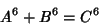(1)

is a special case of Fermat's Last Theorem with, and so has no solution. Ekl (1996) has searched and found no solutions to the 2-2(2)

with sums less than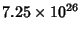.

No solutions are known to the 3-1 or 3-2 equations. However, parametric solutions are known for the 3-3 equation(3)

(Guy 1994, pp. 140 and 142). Known solutions are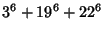(4)(5)(6)(7)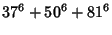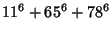(8)(9)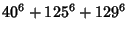(10)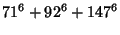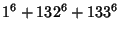(11)(12)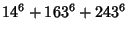(13)

(Rao 1934, Lander et al. 1967).

No solutions are known to the 4-1 or 4-2 equations. The smallest primitive 4-3 solutions are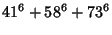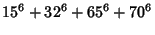(14)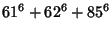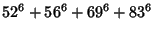(15)(16)(17)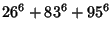(18)

(Lander et al. 1967). Moessner (1947) gave three parametric solutions to the 4-4 equation. The smallest 4-4 solution is(19)

(Rao 1934, Lander et al. 1967). The smallest 4-4-4 solution is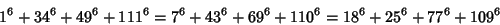(20)

(Lander et al. 1967).

No-1 solutions are known for(Lander et al. 1967). No solution to the 5-1 equation is known (Guy 1994, p. 140) or the 5-2 equation.

No solutions are known to the 6-1 or 6-2 equations.

The smallest 7-1 solution is(21)

(Lander et al. 1967). The smallest 7-2 solution is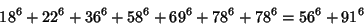(22)

(Lander et al. 1967).

The smallest primitive 8-1 solutions are(23)(24)(25)(26)(27)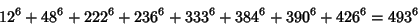(28)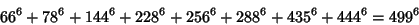(29)(30)(31)(32)(33)(34)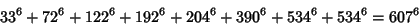(35)(36)

(Lander et al. 1967). The smallest 8-2 solution is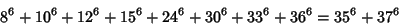(37)

(Lander et al. 1967).

The smallest 9-1 solution is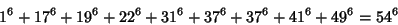(38)

(Lander et al. 1967). The smallest 9-2 solution is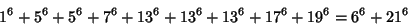(39)

(Lander et al. 1967).

The smallest 10-1 solution is(40)

(Lander et al. 1967). The smallest 10-2 solution is(41)

(Lander et al. 1967).

The smallest 11-1 solution is(42)

(Lander et al. 1967).

There is also at least one 16-1 identity,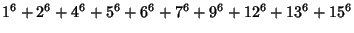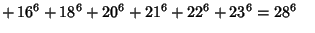(43)
(Martin 1893). Moessner (1959) gave solutions for 16-1, 18-1, 20-1, and 23-1.

References

Ekl, R. L. Equal Sums of Four Seventh Powers.'' Math. Comput. 65, 1755-1756, 1996.

Guy, R. K. Sums of Like Powers. Euler's Conjecture.'' §D1 in Unsolved Problems in Number Theory, 2nd ed. New York: Springer-Verlag, pp. 139-144, 1994.

Lander, L. J.; Parkin, T. R.; and Selfridge, J. L. A Survey of Equal Sums of Like Powers.'' Math. Comput. 21, 446-459, 1967.

Martin, A. On Powers of Numbers Whose Sum is the Same Power of Some Number.'' Quart. J. Math. 26, 225-227, 1893.

Moessner, A. On Equal Sums of Like Powers.'' Math. Student 15, 83-88, 1947.

Moessner, A. Einige zahlentheoretische Untersuchungen und diophantische Probleme.'' Glasnik Mat.-Fiz. Astron. Drustvo Mat. Fiz. Hrvatske Ser. 2 14, 177-182, 1959.

Rao, S. K. On Sums of Sixth Powers.'' J. London Math. Soc. 9, 172-173, 1934.